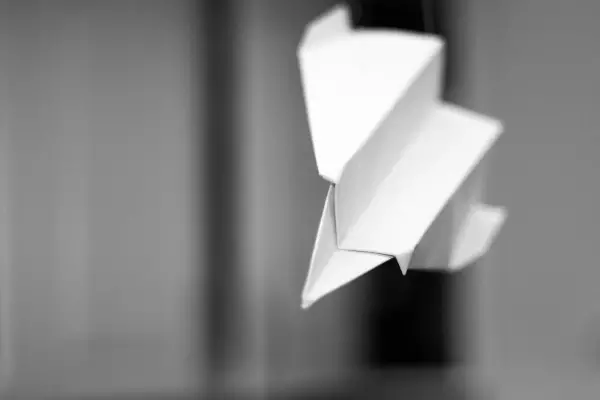# How far would a paper plane thrown from 35000ft fly?

29 September 2015

## Paper plane## Question

If you were able to take a paper airplane and throw it from 35000 ft, how long will it fly and how far will it go?

We put Mo's question to Chris Smith, to do some number crunching...

Kat - That's my level.

Chris - Well then we can use a simple bit of physics to work out how long that would stay airborne for. You need one of these equations of motion. So let's say the equation is d (distance) equals - you do a half times acceleration due to gravity times time (t) squared. So we need to do a bit of rearrangement. So, the distance is 35,000 feet - so that's about 11,000 metres. 11,000 metres equals a half times the acceleration due to gravity: that's 10 times t squared. So we're going to times by two across the board to get rid of the half. That's 22,000 metres equals the acceleration, which is 10 times the speed of gravity, multiplied by the time squared. Now, we're going to divide everything by 10 because the gravity is about 10 metres per second per second. So, we've got about 2,200 equals t squared; the square root of 2,200 is about 50. So, your ball of paper would fall for at least 50 seconds from 35,000 feet. In other words, you'd achieve, even if you'd made the world's worst paper aeroplane, a flight time of a minimum of about a minute.

Andrew- It probably wouldn't actually reach terminal velocity!

Chris - That's not including air resistance I should say!

Andrew - I suspect it would last a lot longer than that!